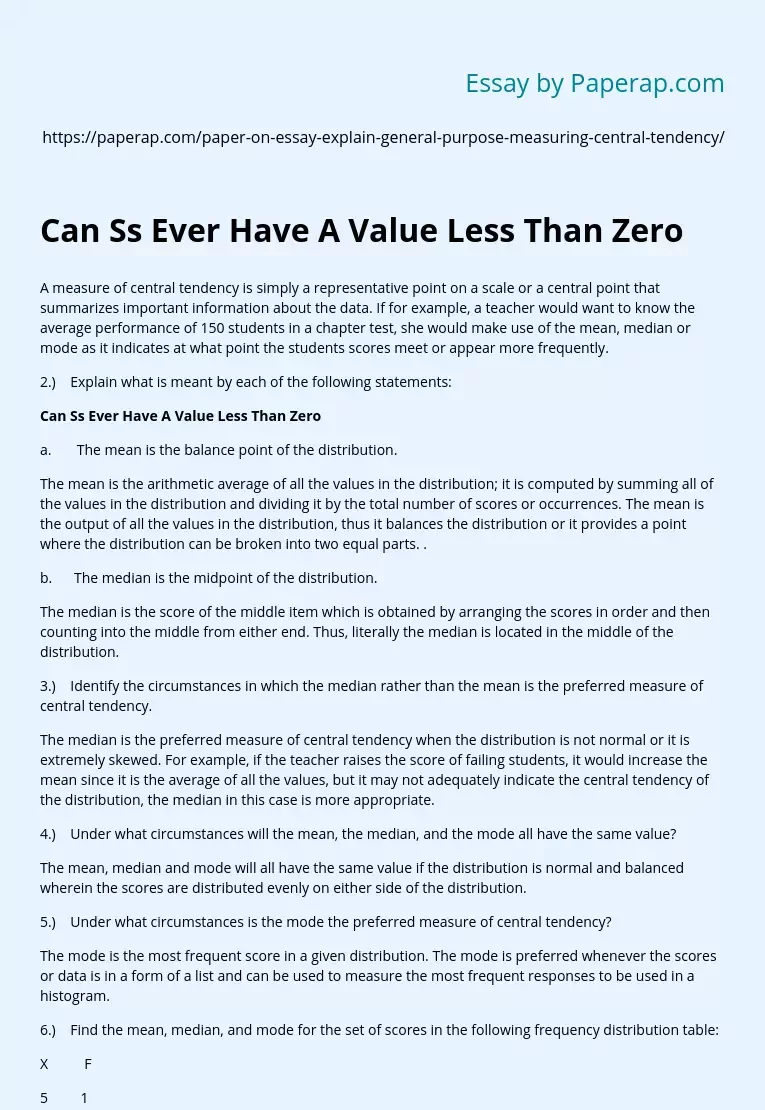# Can Ss Ever Have A Value Less Than Zero

A measure of central tendency is simply a representative point on a scale or a central point that summarizes important information about the data. If for example, a teacher would want to know the average performance of 150 students in a chapter test, she would make use of the mean, median or mode as it indicates at what point the students scores meet or appear more frequently.

2.)    Explain what is meant by each of the following statements:

## Can Ss Ever Have A Value Less Than Zero

a.       The mean is the balance point of the distribution.

The mean is the arithmetic average of all the values in the distribution; it is computed by summing all of the values in the distribution and dividing it by the total number of scores or occurrences. The mean is the output of all the values in the distribution, thus it balances the distribution or it provides a point where the distribution can be broken into two equal parts.

.

b.      The median is the midpoint of the distribution.

The median is the score of the middle item which is obtained by arranging the scores in order and then counting into the middle from either end. Thus, literally the median is located in the middle of the distribution.

3.)    Identify the circumstances in which the median rather than the mean is the preferred measure of central tendency.

The median is the preferred measure of central tendency when the distribution is not normal or it is extremely skewed.

Get quality help nowKarrieWritesVerified

Proficient in: Mathematics5 (339)

“ KarrieWrites did such a phenomenal job on this assignment! He completed it prior to its deadline and was thorough and informative. ”+84 relevant experts are online

For example, if the teacher raises the score of failing students, it would increase the mean since it is the average of all the values, but it may not adequately indicate the central tendency of the distribution, the median in this case is more appropriate.

4.)    Under what circumstances will the mean, the median, and the mode all have the same value?

The mean, median and mode will all have the same value if the distribution is normal and balanced wherein the scores are distributed evenly on either side of the distribution.

5.)    Under what circumstances is the mode the preferred measure of central tendency?

The mode is the most frequent score in a given distribution. The mode is preferred whenever the scores or data is in a form of a list and can be used to measure the most frequent responses to be used in a histogram.

6.)    Find the mean, median, and mode for the set of scores in the following frequency distribution table:

X          F

5         1

4         2

3         3

2         5

1         1

Mean = 2.75

Median = 2.50

Mode = 2.0

7.)    The following frequency distribution summarizes the number of absences for each student in a class of n=20:

Number of

Absences (X)         F

5 or more               3

4                             4

3                             3

2                             6

1                             3

0                             1

a.)    Find the mode for this distribution.

Mode = 6

b.)    Find the median number of absences for this class. Median = 2.50

c.)    Explain why you can not compute the mean number of absences using the data provided in the table.

The data contains a value that cannot be adequately identified; the value 5 or more does not lend itself to the mean because the computation for the mean must consider all of the values that occurred in the distribution.

8.)    In words, explain what is measured by each of the following:

a.)    SS – the sum of squares indicate the sum of the squared differences from the mean.

b.)    Variance – the variance measures how far the scores making up a distribution depart from that distribution’s mean.

c.)    Standard Deviation – the variance is expressed in squared units of measurement and thus it is often misleading, in order to gets the variance using the original units of measurement, the square root of the variance is the standard deviation value.

9.)    A population has u=100 and o=20. If you select a single score from this population, on the average, how close would it be to the population mean? Explain your answer.

The more the scores or cases are in a sample, the more the errors of sampling are reduced, thus for this population I believe that the number I choose would be very close to the population means as the sample has a mea of 100 and a standard deviation of 20.

10.)                       Can SS ever have a value less than zero? Explain your answer.

No, SS cannot have values less than zero for it is derived from the square of the mean differences in the distribution, even if mean difference is negative, once it is squared, the negative sign disappears.

11.)                       What does it mean for a sample to have a standard deviation of zero? Describe the scores in such a sample.

If the standard deviation is zero, it means that the distribution of scores or values are similar or equal, therefore, there is no variation in the scores.

12.)                       What does it mean for a sample to have a standard deviation of s=5? Describe the scores in such a sample. (Describe where the scores are located relative to the sample mean.

When the standard deviation is small, it means that the scores are concentrated near the mean, while standard deviations like more than 30 means that the scores in the distribution are spread out and far from the mean.

13.)                       Calculate SS, Variance, and Standard Deviation for the following population of N=6 scores: 5, 0, 9, 3, 8, 5. (Note: The computational formula for SS works well with these scores.)